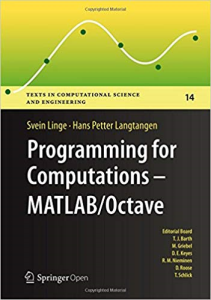Programming for Computations - MATLAB/Octave: A Gentle Introduction to Numerical Simulations with MATLAB/Octave (Texts in Computational Science and Engineering) 1st ed. 2016 Edition

PLDZ-6 In Stock
\$ 6.00 USD
Description

This book presents computer programming as a key method for solving mathematical problems. There are two versions of the book, one for MATLAB and one for Python. The book was inspired by the Springer book TCSE 6: A Primer on Scientific Programming with Python (by Langtangen), but the style is more accessible and concise, in keeping with the needs of engineering students. The book outlines the shortest possible path from no previous experience with programming to a set of skills that allows the students to write simple programs for solving common mathematical problems with numerical methods in engineering and science courses. The emphasis is on generic algorithms, clean design of programs, use of functions, and automatic tests for verification.

Recent Reviews Write a Review
0 0 0 0 reviews